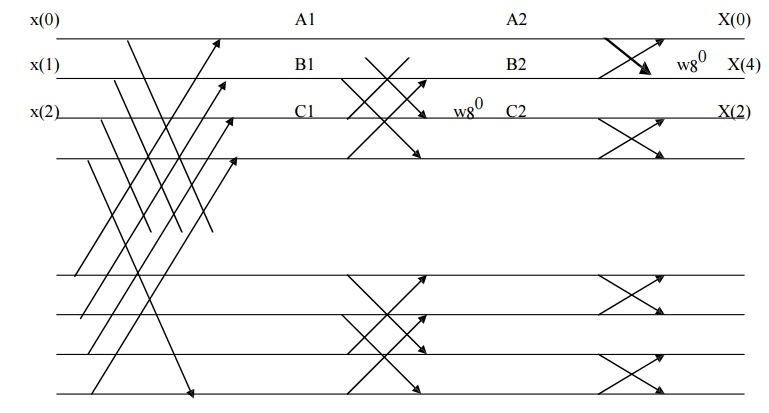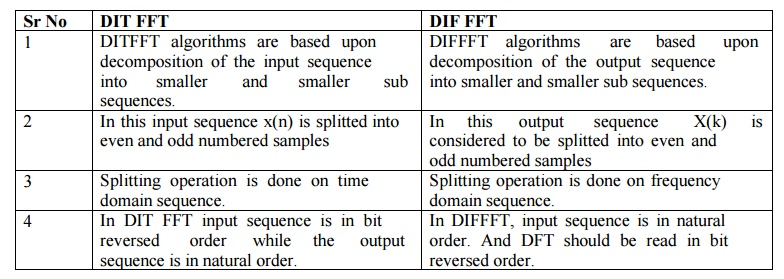Home | | Discrete Time Systems and Signal Processing | Decimation In Frequency (DIFFFT)

# Decimation In Frequency (DIFFFT)

In DIF N Point DFT is splitted into N/2 points DFT s. X(k) is splitted with k even and k odd this is called Decimation in frequency(DIF FFT).

DECIMATION IN FREQUENCY (DIFFFT)

In DIF N Point DFT is splitted into N/2 points DFT s. X(k) is splitted with k even and k odd this is called Decimation in frequency(DIF FFT).

N point DFT is given asSince the sequence x(n) is splitted N/2 point samples, thusLet us split X(k) into even and odd numbered samplesFig 2 shows signal flow graph and stages for computation of radix-2 DIF FFT algorithm of N=4Fig 3 shows signal flow graph and stages for computation of radix-2 DIF FFT algorithm of N=8DIFFERENCE BETWEEN DITFFT AND DIFFFTDIT FFT

1. DITFFT algorithms are based upon decomposition of the input sequence into smaller and smaller sub sequences.

2. In this input sequence x(n) is splitted into even and odd numbered samples

3. Splitting operation is done on time domain sequence.

4. In DIT FFT input sequence is in bit reversed order while the output sequence is in natural order.

DIF FFT

1. DIFFFT algorithms are based upon decomposition of the output sequence into smaller and smaller sub sequences.

2. In this output sequence X(k) is considered to be splitted into even and odd numbered samples

3. Splitting operation is done on frequency domain sequence.

4. In DIFFFT, input sequence is in natural order. And DFT should be read in bit reversed order.

DIFFERENCE BETWEEN DIRECT COMPUTATION & FFTDirect Computation

1. Direct computation requires large number of computations as compared with FFT algorithms.

2. Processing time is more and more for large number of N hence processor remains busy.

3. Direct computation does not requires splitting operation.

4. As the value of N in DFT increases, the efficiency of direct computation decreases.

5. In those applications where DFT is to be computed only at selected values of k(frequencies) and when these values are less than log2N then direct computation becomes more efficient than FFT.

1. Radix-2 FFT algorithms requires less number of computations.

2. Processing time is less hence these algorithms compute DFT very quickly as compared with direct computation.

3. Splitting operation is done on time domain basis (DIT) or frequency domain basis (DIF)

4. As the value of N in DFT increases, the efficiency of FFT algorithms increases.

5. Applications 1) Linear filtering 2) Digital filter design

Q) x(n)={1,2,2,1} Find X(k) using DITFFT.

Q) x(n)={1,2,2,1} Find X(k)using DIFFFT.

Q) x(n)={0.3535,0.3535,0.6464,1.0607,0.3535,-1.0607,-1.3535,-0.3535} Find X(k) using DITFFT.

Q) Using radix 2 FFT algorithm, plot flow graph for N=8.Study Material, Lecturing Notes, Assignment, Reference, Wiki description explanation, brief detail
Digital Signal Processing : Frequency Transformations : Decimation In Frequency (DIFFFT) |